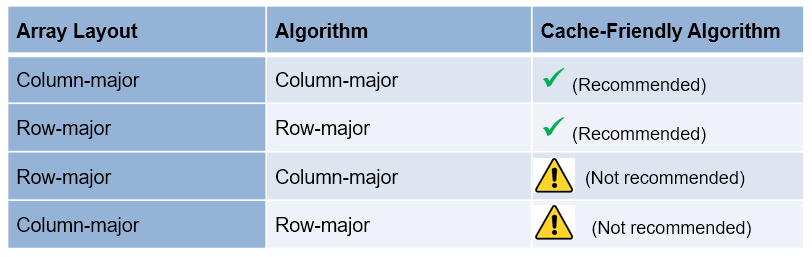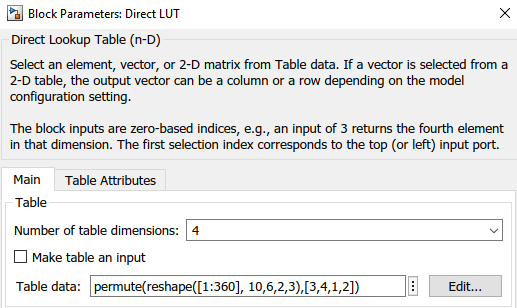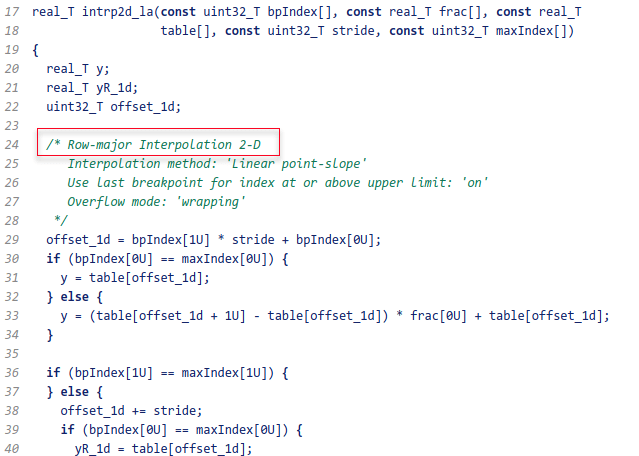# Column-Major Layout to Row-Major Layout Conversion of Models with Lookup Table Blocks

Simulink® Coder™ supports row-major array layout for code generation. You can integrate existing applications that use row-major array layout with the generated code in row-major array layout. When you switch an existing model with lookup table (LUT) blocks from the column-major array layout to the row-major array layout, it is recommended to convert the LUT blocks from the column-major algorithm to the row-major algorithm. The code generated by using row-major algorithm performs with the best speed and memory usage when operating on table data with row-major array layout. The code generated by using column-major algorithm performs best with column-major array layout.

This example shows the workflow of converting a model with LUT blocks from column-major layout to row-major layout to achieve the best performance on a row-major array layout.

In this example, you:

• Identify the array layout and select the optimized algorithm.

• Preserve semantics through table permutation.

• Generate code by using a row-major algorithm and an array layout.

### Simulate and Generate Code by Using Column-Major Algorithms

1. Open the example model `RowLUTColToRow`.

```open_system('RowLUTColToRow'); ```By default, Simulink configures a model to use column-major algorithms and a column-major array layout. These parameters are the configuration parameters in the Configuration Parameters dialog box.2. On the Modeling tab, click Run to simulate the model and observe the output logged in workspace variable `yout`.

3. Change your current folder in MATLAB® to a writable folder. On the C Code tab, click Build to generate C code.

### Select Optimized Algorithms for Row-Major Array Layout

Table data with row-major array layout is frequently used in the field of calibration. To interface the row-major table data with the existing model, update the column-major model to operate efficiently on row-major table data.

Use the algorithm that is optimized for the specified array layout to achieve the best performance. For example, use row-major algorithms when Array layout is set as `Row-major` during code generation.1. To enable row-major algorithms, open the Configuration Parameters dialog box. On the Math and Data Types pane, select the configuration parameter Use algorithms optimized for row-major array layout. Alternatively, in the MATLAB Command Window, enter:

```set_param('RowLUTColToRow','UseRowMajorAlgorithm','on'); ```

2. Click Run to simulate the model. Simulink reports errors because it encounters inconsistent breakpoint and table data between prelookup and interpolation blocks. The causes of this error are the two semantic changes that occur when you switch from column-major algorithms to row-major algorithms, that is, when you:

• Select a plane from a 3-D table in interpolation block.

• Select a plane from a 4-D table through a direct lookup table block.### Preserve Semantics by Using Table Permutation

1. For subtable selection before interpolation, or direct lookup that outputs a vector or 2-D matrix, the model semantics change when you switch from a column-major algorithm to a row-major algorithm by selecting the configuration parameter Use algorithms optimized for row-major array layout. To preserve the semantics and fix the previous errors, permute the table data by using these commands:

```T4d_str = get_param('RowLUTColToRow/Direct LUT','Table'); set_param('RowLUTColToRow/Direct LUT','Table',... ['permute(',T4d_str,',[3,4,1,2])']); ```
```T3d_str = get_param('RowLUTColToRow/Interp2','Table'); set_param('RowLUTColToRow/Interp2','Table',... ['permute(',T3d_str,',[3,1,2])']); ```

2. Before you import table data from a file, you must permute the table data in the file. This permutation keeps the table tunable throughout the simulation and code generation workflow.

### Code Generation by Using Row-Major Algorithm and Array Layout

After permuting the table data, Simulink configures the model `RowLUTColToRow` for row-major simulation. The model is equivalent to the preconfigured model `RowLUTColToRowPreconfigured` that has permuted table data and uses a row-major algorithm.

1. Open the example model `RowLUTColToRowPreconfigured`.

```open_system('RowLUTColToRowPreconfigured'); ```2. To set up these models for row-major code generation, open the Configuration Parameters dialog box. In addition to enabling the Use algorithms optimized for row-major array layout configuration parameter, on the Code Generation > Interface pane, set the configuration parameter Array Layout to `Row-Major` option. The Array Layout parameter enables the model for row-major code generation. Alternatively, in the MATLAB Command Window, enter:

```% For model 'RowLUTColToRowPreconfigured' set_param('RowLUTColToRowPreconfigured', 'ArrayLayout','Row-major'); % For model 'RowLUTColToRow' set_param('RowLUTColToRow', 'ArrayLayout','Row-major'); ```

3. In the block dialog boxes, examine the permuted 3-D table.4. Change your current folder in MATLAB to a writable folder. On the C Code tab, click Build to generate C code. In the generated code, observe the table data with row-major array layoutIn the generated code, the `memcpy` function replaces the `for` loops. Using `memcpy` reduces the amount of memory for storing data. This optimization improves execution speed.Observe the algorithms optimized for row-major data.```close_system('RowLUTColToRow',0); close_system('RowLUTColToRowPreconfigured',0); ```